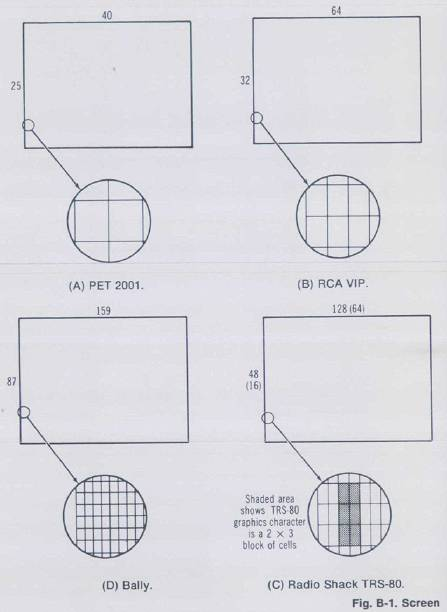Appendix B

Screen Comparisons

This appendix is presented as an aid to revealing and comparing the actual screen densities of the most popular low-cost home computers that feature graphics. In Fig. B-1 the screen outlines are drawn and the numbers of horizontal dot columns and vertical dot rows are labeled. If the dot matrix is indirectly accessible (i.e., no software instruction exists for easily turning a dot on or off) then the indirect resolution is given in parenthesis, and the character resolution is marked on the screen. If mixed graphics is allowed (that is, mixing text or graphics characters with graphics dots) then the character matrix is indicated by heavy lines around the lighter denser matrix.

Under each screen drawing is a circled enlargement of the dot matrix of the computer. These have all been drawn to the same scale so that comparisons can easily be made between them. To create the spacing for the enlargements, it was assumed that for all computers the aspect ratio of the tube was 3:4 and the actual screen dimensions were assumed to be 32 by 24 inches (about 81 by 61 centimeters). The large size was chosen for ease of calculations and doesn't represent a true size of screen. Next the spacing for the horizontal dots was determined by dividing 32 by the number of horizontal dots advertised for the computer, and the vertical spacing by dividing 24 (32X¾) by the number of vertical dots advertised. For example, the 512 horizontal dots of the Exidy Sorcerer, have a 32/512 = 1/16 inch (0.16 cm) spacing, and the vertical spacing is 24/240 = 0.1 inch (0.25 cm).

The screen formats are reduced in the same proportion and are presented in order of increasing screen density.

Notes about the characteristics of the graphics are given when deemed necessary under the screen. The set here is not to be considered exhaustive, but represents a fair sampling of the most popular low-cost graphics-oriented computers.Fig. B-1. Screen density comparisons.